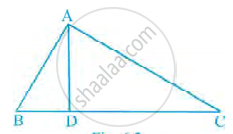# In Figure, ∠BAC = 90° and AD ⊥ BC. Then, ______. - Mathematics

MCQ
Fill in the Blanks

In Figure, ∠BAC = 90° and AD ⊥ BC. Then, ______.#### Options

• BD . CD = BC2

• AB . AC = BC2

• BD . CD = AD2

• AB . AC = AD2

#### Solution

In Figure, ∠BAC = 90° and AD ⊥ BC. Then, BD . CD = AD2.

Explanation:

We have,

∠D = ∠D = 90°  ......(∵ AD ⊥ BC)

∠DBA = ∠DAC  ......[Each angle = 90° – ∠C]

Using AAA similarity criteria,

Concept: Similar Figures
Is there an error in this question or solution?

#### APPEARS IN

NCERT Mathematics Exemplar Class 10
Chapter 6 Triangles
Exercise 6.1 | Q 1 | Page 60
Share